﻿ The Determination of the Solubility Parameter (δ) and the Mark-Houwink Constants (K & α) of Food Grade Polyvinyl Acetate (PVAc)

### The Determination of the Solubility Parameter (δ) and the Mark-Houwink Constants (K & α) of Food...

Ronald P. D’Amelia, Jaksha C. Tomic, William F. NirodeOPEN ACCESSPEER-REVIEWED

## The Determination of the Solubility Parameter (δ) and the Mark-Houwink Constants (K & α) of Food Grade Polyvinyl Acetate (PVAc)

Ronald P. D’Amelia1,, Jaksha C. Tomic1, William F. Nirode1

1Department of Chemistry, Hofstra University, Hempstead, New York

### Abstract

Polyvinyl alkyl ester of carboxylic acids are a family of macromolecules in which the side chain esters (pendant groups) increase in molar mass and hydrophobicity and decrease in structural polarity as the number of carbons in the carboxylic acid increases. The most important polymer in this family is Polyvinyl Acetate (PVAc). The Solubility Parameter (δ) is a unique physical property of any polymeric material and can be a useful guide to understanding the miscibility or compatibility of two polymeric substances. It is therefore essential in working with polymeric blends of PVAc that the experimental solubility parameter be accurately and precisely known. We have experimentally determined the solubility parameter of food grade PVAc by measuring the intrinsic viscosity of several different molecular weight PVAc samples (ranging from 11K -75K Daltons) in four different solvents (acetone, methanol, tetrahydrofuran, toluene,) at 25°C using glass capillary viscometry. We also estimated the solubility parameter using the principles of group additivity contribution due to the atoms, groups and bonds present in PVAc based on the theories of Small, Hoy, and Van Krevelen. The Mark-Houwink constants for PVAC in the four solvents were also experimentally determined. Our experimentally determined solubility parameter was 9.35 (cal/cm3)1/2 which compared well with the computational values obtained by Hoy (9.56), Small (9.45) and Van Krevelen (9.27).

### At a glance: Figures

1
Prev Next

• D’Amelia, Ronald P., Jaksha C. Tomic, and William F. Nirode. "The Determination of the Solubility Parameter (δ) and the Mark-Houwink Constants (K & α) of Food Grade Polyvinyl Acetate (PVAc)." Journal of Polymer and Biopolymer Physics Chemistry 2.4 (2014): 67-72.
• D’Amelia, R. P. , Tomic, J. C. , & Nirode, W. F. (2014). The Determination of the Solubility Parameter (δ) and the Mark-Houwink Constants (K & α) of Food Grade Polyvinyl Acetate (PVAc). Journal of Polymer and Biopolymer Physics Chemistry, 2(4), 67-72.
• D’Amelia, Ronald P., Jaksha C. Tomic, and William F. Nirode. "The Determination of the Solubility Parameter (δ) and the Mark-Houwink Constants (K & α) of Food Grade Polyvinyl Acetate (PVAc)." Journal of Polymer and Biopolymer Physics Chemistry 2, no. 4 (2014): 67-72.

 Import into BibTeX Import into EndNote Import into RefMan Import into RefWorks

### 1. Introduction

Polyvinyl alkyl ester of carboxylic acids are a family of macromolecules in which the side chain esters (pendant groups) increase in molar mass and hydrophobicity and decrease in structural polarity as the number of carbons in the carboxylic acid increases. The most important polymer in this family is Polyvinyl Acetate (PVAc). It is usually produced by the free radical polymerization of vinyl acetate either in a non-aqueous solvent or as an emulsion. PVAc is an extremely important commercial polymer used as the major polymeric component in some water-based latex paints, wood adhesives, paper binders and as a food masticatory component in chewing and bubble gums. PVAc is also the precursor for making Polyvinyl Alcohol (PVOH), the single largest volume water-soluble polymer in the world.

PVAc is considered a thermoplastic, atatic, amorphous, non-toxic polymer whose bulk properties range from a gummy solid at low molecular weight to a brittle leathery material at high molecular weight. Understanding the physical and chemical properties of PVAc is essential in understanding its functional uses in bulk, solution or in a commercial product. The solubility parameter (δ) is an unique physical property of any polymeric material and can be a useful guide to understanding the miscibility or compatibility of two polymeric substances. It is therefore essential in working with polymeric blends of PVAc that the solubility parameter be accurately and precisely known.

Burrell  developed a method of determining the solubility parameter of a polymer by measuring the solution properties in a series of solvents and then relating its solubility parameter to that of the solvent in which the polymer is soluble in all proportions. Gee  also measured the solubility parameter by determining the degree of swelling of cross-linked polymers in various solvents, but difficulties were presented because of the poor sensitivity of the measuring technique. Mangaraj et. al.  estimated the cohesive energy density of various polymers of polyacrylates and polymethacrylates using viscosity measurements. Books, articles and comprehensive reviews of the theoretical and practical aspects of measuring the solubility parameter, intrinsic viscosity and the Mark-Houwink relationship of polymers have been reported [4-15]. Also, there are numerous references of determining the solubility parameter and the Mark-Houwink properties of PVAc [16-22]. Theoretical methods based on the principles of group additivity contributions due to the atoms and groups and bonds present in a polymer have also been developed in order to evaluate the solubility parameter and other properties of polymers [23, 24, 25, 26].

Our research entailed experimentally determining the solubility parameter (δ) of PVAc by measuring the intrinsic viscosity [η] of several different molecular weight PVAc samples in four different solvents (acetone, methanol, tetrahydrofuran (THF), and toluene,) at 25°C using glass capillary viscometry. Glass capillary viscometry is a technique used to experimentally determine the dilute solution intrinsic viscosity of a solution. This method determines the flow times of a series of dilute polymer solutions and pure solvent using a capillary viscometer and calculation of intrinsic viscosity. The intrinsic viscosity or limiting viscosity number (LVN) is a viscosity number extrapolated to infinite dilution. It is a measure of the ability of an isolated polymer molecule to increase the viscosity of the solvent in absence of intermolecular interactions between polymer molecules. We can use this technique to evaluate the solubility parameter because theory predicts that a maximum value in the intrinsic viscosity in a specific solvent corresponds to the solubility parameter of the individual macromolecule. We computationally determined the solubility parameter (δ) for PVAc using the semi-empirical group additivity theory based on the models of Small [23, 24], Hoy , and Van Krevelen . Based on our experimentally determined intrinsic viscosity and the weight average molecular weight (Mw) by light scattering, the Mark-Houwink constants for PVAc for each solvent were also determined.

### 2. Materials and Methods

2.1. Sample Description

All samples were produced by the Union Carbide Corp (now part of the Dow Chemical Co.) by the solution free radical polymerization of vinyl acetate. They are all clear, water white, food grade resins with very little residuals. Samples were identified as: GB-11, GB-30, GB-50, GB-75. All these samples of PVAc are 99+% atatic amorphous homopolymers.

2.2. Glass Capillary Dilute Solution Viscometry

The experimental objective was to obtain the solution viscosities of four different samples of PVAc in four different solvents: acetone, methanol, toluene and THF. Viscometry measurements were made using a calibrated Cannon-Ubbelohde low shear semi-micro dilution viscometer (size 50). The temperature was controlled using a Cannon constant temperature bath maintained at 25.0°C ± 0.1°C. Triplicate readings were taken at each concentration using a stopwatch accurate to 0.1 sec. The intrinsic viscosity data as well as the Huggins  and Kraemer  constants were obtained by extrapolating to zero concentration a plot of reduced specific viscosity [viscosity number] (ηsp/c) and inherent viscosity [logarithmic viscosity number] (ln ηrel/c) vs concentration in g/dl. The intersection of the Kraemer  and Huggins  plots was taken as the intrinsic viscosity [η].

2.3. Specific Refractive Index Increment

The experimental objective was to obtain the dn /dc values of the four samples of PVAC in the same four solvents. A Wyatt Optilab model DSP interferometer refractometer having a P-10 cell maintained at 25.0°C. The dn /dc values were obtained at 690 nm. The instrument was calibrated using aqueous NaCl solutions. The data was analyzed from plots of Δn vs concentration using Wyatt DNDC software. The slopes of the Δn vs concentration plots were the dn/dc values for each solution. The values of dn/dc were used in conjunction with light scattering data to obtain Mw.

2.4. Light Scattering Study

The objective of the light scattering study was to determine the Weight Average Molecular weights (Mw) and solvent-solute interaction parameters (A2) of the samples of PVAc in THF at 25°C using Multi Angle Laser Light Scattering (MALLS) Photometry. A Wyatt MALLS photometer model mini DAWN containing a K5 cell was used. The scattering intensities were measured at three angles (45°, 90°, and 135°) using 690 nm wavelength of light. The data was analyzed using Zimm plots obtained from double extrapolated plots of Kc/Rθ vs Sin2 θ/2 + kc using Wyatt Aurora software.

2.5. Theory
2.5.1. Fundamental Principles of Dilute Solution Viscometry

The viscosity (η) of a substance is defined as the force per unit area required to maintain a unit velocity gradient between two parallel plates kept at a constant distance apart. Viscosity can simply be described as the degree to which a fluid resists flow under an applied force. The use of a glass capillary viscometer has provided a simple and easy technique for measuring the viscosity of dilute polymeric solutions. The principle of this method is that the polymeric solution flows by gravity through a given length of capillary and the time (t) taken for a given volume to pass through (called efflux time) is compared to the time (to) for the pure solvent. The relative viscosity (ηr) also referred to as the viscosity ratio is expressed as shown in equation (1)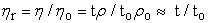(1)

where η is the viscosity, ρ is the density of the solution and ηo and ρo are the viscosity and density, respectively, of the pure solvent. Usually, ρ and ρo for very dilute solutions are nearly equal so that ηr = t/to is a very good approximation. The specific viscosity of the solution (ηsp) is calculated as the difference between viscosities of the solution and the solvent, expressed as a fraction of the viscosity of the solvent as shown in equation (2)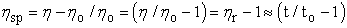(2)

Since viscosity varies with the concentration of the solute, c, a quantity called reduced viscosity (also called the viscosity number), ηred, expresses the specific viscosity per unit of concentration as shown in equation (3)(3)

In the above equation concentration is conventionally expressed in grams per deciliter. Also related to concentration is inherent viscosity (also called logarithmic viscosity number), ηinh as shown in equation (4)(4)

By extrapolating the plot of both the reduced viscosity (ηred) and inherent viscosity (ηinh) to zero concentration gives the intrinsic viscosity ([η]) (or the limiting viscosity number, LVN) as shown in equation (5)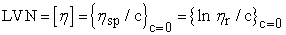(5)

To determine intrinsic viscosity, viscosity data is measured at several concentrations and the [η] is obtained by applying both the Huggins (eq. 6) and Kraemer (eq. 7) equations as shown below:(6)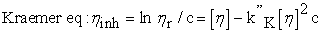(7)

Where kH and kK are dimensionless constants for a series of polymers of different molecular weights in a given solvent and temperature and when added together ≈ 1/2. In both forms of the equation, [η] is the intercept, so plotting either the reduced or the inherent viscosity against concentration will give the same result on extrapolation to zero concentration (infinite dilution). Since k” is usually negative and smaller in magnitude than k’, the inherent viscosity will change less rapidly with concentration than the specific viscosity.

When the log of the intrinsic viscosities of a series of linear polymer homologs of varying molecular weights are plotted against the log of their molecular weights, a linear relationship in the form of the Mark-Houwink equation is obtained as shown in equation (8)(8)

Where, K and α are constants determined, respectively, by the intercept and the slope of the double log plot of log [η] vs log Mw. It has been observed that the exponent, α, does not fall below 0.5 in any case and seldom exceeds about 0.80 for typical linear amorphous synthetic polymers. Once K and α have been established for a given polymer in a given solvent at a specific temperature, molecular weights maybe determined from intrinsic viscosities measurements alone rather than more complicated methods.

2.5.2. Fundamental Principles of Light Scattering Data

One method that is especially useful in calibrating intrinsic viscosity in terms of molecular weight is light scattering. Light scattering is an absolute method of determining the weight average molecular weight of many synthetic polymers above the molecular weight of about 10K daltons. Polymer molecules having a size much less than the wave length used in the experiment can be considered a point source of scattered light and the extent of scattering will vary according to their concentration as expressed in the Dedye virial equation (9)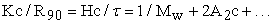(9)

In this equation K and H are light scattering constants, c is the concentration, τ is the total light scattering expressed as turbidity, Mw is weight average molecular weight, and A2 is the second virial coefficient. The light scattering calibrating constants, K and H are determined as shown in eqs 10 and 11, where n and no are the refractive indices of the solution and the solvent, respectively, No is Avogadro’s number, and λ is the wave length of light at which the measurement is made. The total light scattering is usually determined by measuring the intensity (R90) of light scattered at 90° to the incident beam.(10)(11)

Equation 9 forms the basis of the determination of polymer molecular weights by light scattering. Beyond the measurement of τ or R90, only the refractive index and the specific refractive index increment (dn/dc) require experimental determination. The dn/dc factor is a constant for a given polymer, solvent and temperature, which is measured with a differential refractometer.

When the macromolecules in solution are larger than 1/20th of the wave length of light, they no longer are considered point sources. Light scattered from one part of the molecule will interfere with light scattered from another part. In other words, turbidity is reduced and scattering depends upon the shape and size of the macromolecules. To correct this condition one employs the relationship shown in equation 12, in which P(θ) is a particle scattering function. This factor is equal to one at zero angle (θ = 0).(12)

Light scattering measurements are taken at a series of concentrations and scattering angles, then are plotted as a rectilinear grid called a Zimm plot allowing extrapolation to both c = 0 and θ = 0, where P(θ) =1 A typical Zimm plot has the y axis as K c/ R(θ) and the x axis as sin2(θ/2) + a scaling factor k, chosen for spreading out the data times concentration, c. From the Zimm plot, the double extrapolation of the plot gives the intercept as the reciprocal of Mw and the slope of the zero angle line as the second virial coefficient whereas the slope of the zero concentration line as function of the angle gives the average radius of gyration.

2.5.3. Fundamental Principles of Solubility Parameter

The thermodynamic equation describing the solution process of an amorphous polymer in a solvent is given by the following classical equation (Eq.13);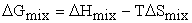(13)

Where: ΔGmix is the change of free energy of mixing; ΔHmix is the change in enthalpy of mixing; T is the absolute temperature; and ΔSmix is the change of entropy of mixing. A negative ΔGmix predicts that the dissolving or mixing process will occur.

The free energy of mixing, ΔGmix, is also given by the following Flory-Huggins equation (14)(14)

Where R is the gas constant; T is the absolute temperature; N1 and N2 are the number of moles of solvent and polymer, respectively; θ1 and θ2 are the volume fraction of solvent and polymer; and χ is the Flory-Huggins interaction parameter. The first two terms of the above equation represent the entropy of mixing and is always negative in this expression. The third term can have a very small positive or negative value in order to obtain a negative free energy of mixing (ΔGmix). The Flory-Huggins polymer-solvent interaction energy (χ) is considered a semi-empirical parameter consisting of both entropic and enthalpic contributions. The enthalpic contribution is given by the following equation (15)(15)

Where V1 is the molar volume of the solvent and δ1 & δ2 are the solubility parameters of the solvent and polymer respectively.

Based on the Hilderbrand-Scott theory the enthalpy of mixing for a polymer-solvent system is given by the following equation (16);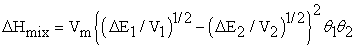(16)

Where Vm is the total volume of the mixture; ΔE1 and ΔE2 are the energies of vaporization of the solvent and polymer respectively; V1 & V2 equals the molar volume of solvent and polymer, respectively and θ1 & θ2 are the volume fractions of solvent and polymer respectively. The term ΔE/V is called the cohesive energy density and is the energy of vaporization per mole. It is a measure of the amount of energy needed to overcome all intermolecular forces. The square root of the cohesive energy density is called the solubility parameter (δ) and has been related as shown in the equation (17) below and is given in units of (cal/cm-3) ½ in the cgs system or H for Hildebrand;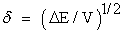(17)

As a general rule the best solvent for a given polymer is one whose solubility parameter is equal or very close to the solubility parameter of the solvent, then ΔHmix and the Flory-Huggins polymer-solvent interaction parameter (χ) equals zero and polymer dissolution occurs.

The solubility parameter for non-polar solvents can easily be determined from its enthalpy of vaporization (ΔHv), density (d) and molar mass (M) by using the following equation (18);(18)

However for non-volatile substances like polymers the solubility parameter cannot be determined directly. The solubility parameter for polymers is usually defined as having the same value as the solvent in which the polymer will dissolve in all proportions without any change in volume or the enthalpy of mixing and would have the highest value for the intrinsic viscosity. If one plots the [η ] values against the solubility parameter of the solvents used in the dilute solution viscosity experiment, the maximum value obtained would correspond to the solubility parameter of the polymer.

2.5.4. Basic Concepts from the Group Additivity Principle

A powerful tool in the semi-empirical approach in the study of the physical properties of polymers is the use of the additivity principle. This principle uses addition or summation of either atomic, group or bond contributions to calculate the molar property of the molecule. This idea is expressed in the equation (19)(19)

Where F is a molar property like solubility parameter, ni is the number of contributing components of the type i, and Fi is the numerical contribution of the component i. Because of the uniqueness of polymers the application of the additivity principle is ideal. The molar quantities are usually expressed per mole of the structural unit.

Small has published  a table of molar attraction constants which allows the estimation of the δ from the structural formula of the polymer and its density. Small data can be found in the Polymer or CRC handbooks. The molar attraction constants G are additive over the formula and are related to the solubility parameter by the following equation (20)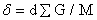(20)

where, ∑G is the sum of the molar attraction constants for all the atoms and groups in the polymer, d is the density and M equals the molar mass of the repeat unit. Hoy has also determined the solubility parameter again using values for molar attraction constants he has determined. His values differ from Small’s values and are shown in the polymer handbook. Hoy uses the following equation to determine the solubility parameter by using the group additivity principle as expressed in equation (21);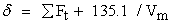(21)

where Vm is the molar volume found by dividing the molecular weight by density. VanKrevlen uses cohesive energy values to compute the solubility parameter using his data and the following equation (22);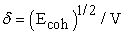(22)

### 3. Results and Discussion

#### Table 1. Summary of Physical Properties for the Solvents

Table 1 summarizes the physical properties of the four solvents used in this study. Using the enthalpy of vaporization (ΔHovap) and Eq. 18 the solubility parameter (δ) was calculated for each solvent used (see Table 2). The calculated values were compared with literature values and were in excellent agreement (see Table 2).

#### Table 2. Summary of the Solubility Parameters for the Solvents

The intrinsic viscosities of the PVAc solutions were obtained by plotting ηred (using the Huggins equation given by Eq. 6) and ηinh (using the Kraemer equation given by Eq. 7) vs concentration of solution in g/dl and extrapolating to zero concentration. The intersection of the intercept of the Huggins and the Kraemer lines was the intrinsic viscosity ([η]) of one of the molecular weight PVAc samples in a specific solvent. A [η] plot of PVAc GB-50 in THF is shown in Figure 1. Similar plots were determined for each of the four samples of PVAc (GB-11, GB-30, GB-50 and GB-75) in each of the four solvents used (acetone, methanol, tetrahydrofuran (THF), and toluene). A summary of the intrinsic viscosity data for each PVAc sample in each solvent studied is presented in Table 3. For each PVAc sample studied, the intrinsic viscosity was found to decrease from THF > Acetone > Toluene > Methanol. Also, the intrinsic viscosity of PVAc increases with increase in molecular weight from GB-11 <, GB-30 <, GB-50 <, GB75.

Figure 1. Intrinsic Viscosity Plot of ηred and ηinh vs Concentration for PVAc GB-50 in THF is shown. Extrapolation of the Huggins (ηred) and the Kraemer (ηinh) lines gives the intrinsic viscosity for the polymer

#### Table 3. Summary of Intrinsic Viscosity Data

A plot of the [η] obtained for each PVAc sample in each solvent studied versus the solubility parameter found in Table 2 was done and shown in Figure 2. For each molecular weight PVAc polymer, the peak shown in Figure 2 corresponds to the maximum intrinsic viscosity obtained at the solubility parameter for the solvent (THF) that is best for the polymer. The solubility parameter for THF was determined to be 9.35 (cal/cc)½.

Using the semi-empirical methods of group additivity from Small, Hoy, and Van Krevelen the δ for PVAc was calculated. These computational values along with the experimental value determined from intrinsic viscosity measurements are summarized in Table 4. The experimentally determined solubility parameter of PVAc value of 9.35 agreed exceptionally well with the literature value of 9.4 from the Polymer Handbook.

Figure 2. Plot of [η] vs solubility parameter (δ) for GB-11, GB-30, GB-50, and GB-75 are shown. The peak in each plot corresponds to the maximum intrinsic viscosity in THF

#### Table 4. Summary of the Solubility Parameter for Food Grade PVAc

A summary of the weight average molecular weights and the second virial coefficient for the four PVAc samples studied in THF at 25°C are shown in Table 5. These were determined from light scattering experiments (see section 2.5 B). The second virial coefficient (A2) decreased as the molecular weight increased.

#### Table 5. Weight Average Molecular Weights (Mw) and Second Virial Coefficients (A2) of each PVAc resin in THF

Figure 3. A double log plot of [η] vs Mw is shown. The slope is equal to the α and the intercept is equal to K, constants of the Mark Houwink equation

Figure 3 shows a double log plot of [η] versus Mw using the data from Table 5. From the slope and intercept from this plot, the Mark-Houwink constants of α and K respectively were determined. These Mark-Houwink constants are summarized in Table 6 and show that α increased and K decreased from methanol, toluene, acetone, THF.

### 4. Conclusions

We have experimentally determined the solubility parameter of food grade PVAc by measuring the intrinsic viscosity of several different molecular weight PVAc samples (ranging from 11K -75K Daltons) in four different solvents (acetone, methanol, tetrahydrofuran, toluene,) at 25°C using glass capillary viscometry. The solubility parameter of PVAc was determined using the principles of group additivity contribution due to the atoms, groups and bonds present in PVAc based on the theories of Small, Hoy, and Van Krevelen. Our experimentally determined solubility parameter was 9.35 (cal/cm3)1/2 which compared well with the computational values obtained by Hoy (9.56), Small (9.45) and Van Krevelen (9.27). The Mark-Houwink constants for PVAc in the four solvents were also experimentally determined.

### Acknowledgments

We acknowledge the support from a Hofstra University HCLAS faculty and research development grant.

### References

  Burrell, H., Off. Dig. Fed. Paint. Varn. Prod., 27, 726, 1955.In article  Gee, G., “Swelling and Solubility in Mixed Liquids”, Trans. Faraday Soc., 40, 468-480, 1944.In article CrossRef  Mangaraj, D., Patra, S., Roy, P.C., “Cohesive Energy Densities of High Polymers: V- C.E.D. of Polyacrylates”, Makromol. Chem., 81, 173-175, 1965In article CrossRef  Burrell, H. Solubility Parameter Values, Polymer Handbook, 2nd ed, (eds Brandrup & Immergut), J. Wiley, 1975, IV: 337-359.In article  Kurata, M., Tsunashima, Y., et. al., Viscosity – Molecular Weight Relationships & Unperturbed Dimensions of Linear Chain Molecules, Polymer Handbook 2nd ed., (eds. Brandrup & Immergut), J.Wiley, 1975, IV: 1-60.In article  Barton, A. F. M., CRC Handbook of Polymer-Liquid Interaction Parameters and Solubility Parameters, CRC Press, Boca Raton, 1990, 297-342.In article  Hansen, C.M., CRC Handbook – Hansen Solubility Parameters: A User’s Handbook, 2nd edition, CRC Press, Boca Raton, 2007, 1-33In article CrossRef  Flory P. J., Principles of Polymer Science, Wiley-Interscience,. Cornel University Press, Ithaca, N.Y 1953, chapters 12 & 13.In article  Billmeyer, F., Textbook of Polymer Science, Wiley-Interscience, New York, 1966, chapter 3E.In article  Rabek, J.F., Experimental Methods in Polymer Chemistry, J. Wiley, New York, 1980, chapter 9, 123-140.In article  Masuelli, M.A., “Mark-Houwink Parameter for Aqueous-Soluble Polymers & Biopolymers at Various Temperatures”, Journal of Polymer & Biopolymer Physics, 2, 2, 37-43, 2014.In article  Chee, K.K., “Huggins’ Constant and Unperturbed Parameter of Dilute Polymer Solutions”, J. Applied Polym. Sci., 27, 5, 1675-1680, 1982.In article CrossRef  Braun, J. L., Kadia, J.F., “A Relative Simple Method for Calculating Mark-Houwink Parameters using Basic Definitions”, J. of Applied Polym. Sci., 114, 5, 3303-3309, 2009.In article CrossRef  Huggins, M.L. “The Viscosity of Dilute Solutions of Long-Chain Molecules, IV- Dependence on Concentration”, J. Am. Chem. Soc. 64, 11, 2716-2718, 1942.In article CrossRef  Kraemer, E.O., Ind. Eng. Chem., 30, 1200, 1938.In article CrossRef  Chinai, S.N., Scherer, P.C. and Levi, D.W.,” Molecular Weight pf Polyvinyl Acetate by Light Scattering and Viscosity Techniques”, J. Polym. Sci. 17 117-124, 1955.In article CrossRef  DiPaola-Baranyi, G., Guillet, J.E., Klein, J. Jeberien, H.W., “Estimation of the Solubility Parameters for Poly (vinyl acetate) by Inverse Gas Chromatography”, J. of Chromatogr. A, 166, 2, 349-356, 1978.In article CrossRef  Fernandez-Berridi, M.J., Guzman, G.M., Elorza, J.M., Garijo, L., “Study by Gas-Liquid Chromatography of the Thermodynamics of the Interaction of Poly (vinyl acetate) with various Solvents”, Eur. Polym. J., 19, 5, 445-450, 1983.In article CrossRef  Daoust, H, Rinfret, M.,” Solubility of Polymethymethacrylate and Polyvinylacetate”, J. Colloid Si., 7, 1, 11-19, 1952.In article CrossRef  Wagner, R. H., “Intrinsic Viscosities and Molecular Weights of Polyvinylacetates”, J. Polym. Sci. 2, 1, 21-35, 1947.In article CrossRef  Merk, W., Lichtenthaler, R. N., Prausnitz, J.M., “Solubilities of Fifteen Solvents in Copolymers of Poly(vinyl acetate) and Poly(vinyl Chloride) from gas Chromatography: Estimation of Polymer Solubility Parameters”, J.Phys. Chem, 84, 13, 1694-1698, 1980.In article CrossRef  Lindermann, M.K. Physical Constants of Polyvinyl Acetate, Polymer Handbook 2nd ed, (eds Brandrup & Immergut), J. Wiley 1975 V51-55.In article  Small, P.A. J. Appl. Chem, 3, 71, 1953.In article CrossRef  Scatchard, G. Chem Rev, 8, 821, 1931.In article CrossRef  Hoy, K.L. J. Paint Technol., 42, 76, 1970.In article  Van Krevelen, D.W., Properties of Polymers:Their Estimation and Correlation with Chemical Structures, Elsevier 2nd ed, 1976.In article  CRC Handbook of Chemistry and Physics 67th ed.; Weast, R.C. Eds,: CRC Press, Boca Raton, Fl., 1986.In article